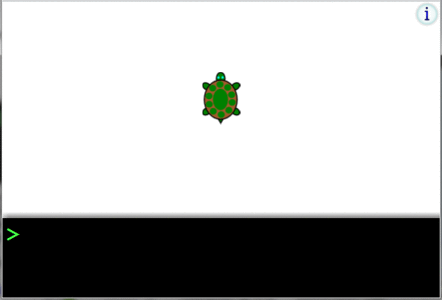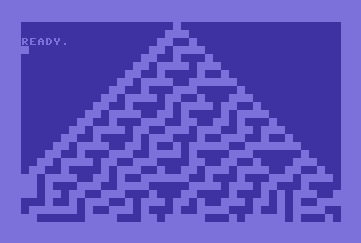Wilfried Elmenreich

## Wilfried Elmenreich

Professor of Smart Grids

# Coding is fun!

Here you will find the results from some programming projects I did in my free time. So, typically they are not related to my work, but some projects have become "research toys", that is a nice thing to play with while also giving insight and contribution to research topics.

## Parsing command line arguments in Java

Although there exist numerous packages for parsing command line options with Java, I wanted to have something compact, where I don't have to link a jar library to my project every time I just want to write a program that parses three command line options. Inspired by this code, I came up with the following simple code structure to do it:

```static void printUsage() { System.out.println("Usage: java main.Main [-a nn] [-xn] file"); } public static void main(String[] args) { int optind = 0; String optarg; while (optind < args.length && args[optind].startsWith("-")) { optarg = args[optind++]; // usage message upon help if (optarg.equals("-?") || optarg.equals("-help") || optarg.equals("--help")) { printUsage(); System.exit(0); } // an option with an integer argument else if (optarg.equals("-sd") || optarg.equals("-seed") || optarg.equals("--seed")) { if (optind < args.length) { //Integer.parseInt(args[optind++]); } else { System.err.println("option requires an argument"); System.exit(1); } } // a series of flag arguments else { for (int j = 1; j < arg.length(); j++) { char flag = arg.charAt(j); switch (flag) { case 'x': System.out.println("Option x"); break; case 'n': System.out.println("Option n"); break; default: System.err.println("ParseCmdLine: illegal option " + flag); break; } } } else { System.err.println("Unknown option: "+optarg); printUsage(); System.exit(1); } } //further arguments while(args.length>optind) System.out.println("further argument:"+args[optind++]); return 0; } ```

## Logo turtle graphics

Logo is a venerable functional programming language (created 1967) which gained most of its popularity from the concept of Turtle Graphics: A small triangle on the screen (the turtle) being directed by simple commands like forward, backward, left, and right, each command followed by an argument giving the distance or turning angle. In combination with the repeat command you could create beautiful drawings with a few commands. Being that simple, the turtle graphics of Logo is a nice way to teach the concept of programming to kids, possibly even pre-school. The following image shows a short animation of a browser-based Turtle Graphics interpreter aimed at introducing kids to computer programming. I extended the original program from John Villar by a few features. Feel free to click on it and play around!## Commodore 64 calculates one-dimensional binary cellular automata

Were the homecomputers of the mid 80ies good for doing any practical work? For example, would they have been useful in doing research on complex systems? I think, the answer is yes! As a proof of concept, I have quickly implemented a short program in Commodore Basic V2.0 that lets you explore the behavior of binary cellulary automata specified by Wolfram's code. Wolfram's work dates also back to the 1980ies, but has recently gained much attention due to his book A new kind of science.

```100 DIM K(8) 110 INPUT"ENTER RULE NUMBER";R 120 N=1 130 FOR I=1 TO 8 140 IF R AND N THEN K(I)=1 150 N=N*2 160 NEXT 170 INPUT"USE RANDOM LINE AS SEED";A\$:RN=0:IF A\$="Y" THEN RN=1 300 PRINT CHR\$(147) 310 IF RN=0 THEN POKE 1043,160:GOTO 340 320 FOR I=1024 TO 2023:IF RND(0)<0.5 THEN POKE I,160 330 NEXT 340 FOR L=1024 TO 1944 STEP 40 350 A=0:IF PEEK(L+39)=160 THEN A=4 360 B=0:IF PEEK(L)=160 THEN B=2 370 FOR P=0 TO 39 380 C=0:IF PEEK(L+1+P)=160 THEN C=1 385 IF P=39 THEN C=0:IF PEEK(L)=160 THEN C=1 390 IF K(A+B+C+1) THEN POKE L+P+40,160 400 A=B*2:B=C*2 410 NEXT 420 NEXT ```

The program displays the results of a simulation block code on the 1000 character 64 screen. Below is the result of simulating the famous rule 30.More about home computers can be found here.

## Calculating the binominal coefficient (n over k)

While the formula for the binominal coefficient is trivial, its implementation can be tricky: (n over k) = n! / (k! * (n-k)!) suggests a straightforward implementation making factorial calculations for n, k, and n-k and then dividing the results. However, this approach comes with a lot of unnecessary multiplications and divisions, and, worse, leads to very high intermediate results even for low numbers. For example, 15! or larger cannot be properly represented within a Java integer variable and even when using long, the maximum n can be only 21.

The following implementation (the syntax works for C, C++ and Java) does better by avoiding unnecessary multiplications and can calculate the exact result of the binominal coefficient up to an n of 61 in Java (and arbitrary k):

```long noverk(int n, int k) { long noverk=1; int i; if (2*k < n) k=n-k; for(i=1;i<=n-k;i++) { noverk *= (i+k); noverk /= i; } return noverk; } ```

## Solar system simulation in Java ScriptThis is a physical simulation of the motion of objects with respect to their mass and Newton's Law of Gravity. The program is written in Javascript and simulates the movement several objects (sun, the eight planets, pluto, and the earth moon) of our solar system. This is the result of a Javascript programming exercise.

To the simulation! (Zoom out for the outer planets. Increasing the simulation speed means using a more coarse-grained resolution. Increase it too much and strange things will happen :-)

## bib2xhtml 2.27

bib2xhtml is an open source tool by David Hull and Diomidis Spinellis for creating a list of references for a webpage. I used this tool also to create the publications list on this page. I extended the previous version 2.26 with a new export type and added a setting that avoids problems with some perl setups (especially under Windows). For details see the changelist included in the archive.